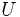# Locally analytic function

## Definition

### At a point

A function$f$ of one variable is said to be locally analytic (or sometimes simply analytic) at a point$x_0$ in the interior of its domain if it satisfies the following equivalent conditions:

1. There exists a (unique) power series centered at$x_0$ that converges to$f$ on an interval of positive radius centered at$x_0$.
2. The Taylor series of$f$ at$x_0$ converges to$f$ on an interval of positive radius centered at$x_0$.

Note that (1) and (2) are equivalent in the following sense: if there is a power series centered at$x_0$ that converges to$f$ on an interval of positive radius centered at$x_0$, that power series must equal the Taylor series.

### On a subset of the domain

A function$f$ of one variable is said to be locally analytic (or sometimes simply analytic) on an open subset$U$ of the domain if it is locally analytic at every point of$U$.

If the open subset is the whole domain, we may simply say that$f$ is locally analytic.